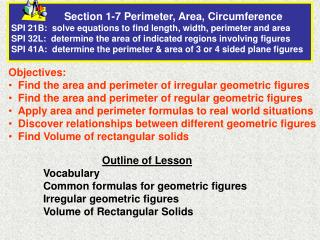DownloadDownload PresentationObjectives: Find the area and perimeter of irregular geometric figures

# Objectives: Find the area and perimeter of irregular geometric figures

Télécharger la présentation## Objectives: Find the area and perimeter of irregular geometric figures

- - - - - - - - - - - - - - - - - - - - - - - - - - - E N D - - - - - - - - - - - - - - - - - - - - - - - - - - -
##### Presentation Transcript

1. Section 1-7 Perimeter, Area, Circumference SPI 21B: solve equations to find length, width, perimeter and areaSPI 32L: determine the area of indicated regions involving figuresSPI 41A: determine the perimeter & area of 3 or 4 sided plane figures • Objectives: • Find the area and perimeter of irregular geometric figures • Find the area and perimeter of regular geometric figures • Apply area and perimeter formulas to real world situations • Discover relationships between different geometric figures • Find Volume of rectangular solids Outline of Lesson Vocabulary Common formulas for geometric figures Irregular geometric figures Volume of Rectangular Solids

2. Vocabulary • Perimeter: • the sum of the lengths of the sides of a polygon • fence around a plot of land • Circumference: • the distance around the outside of a circle • the distance around the earth

3. Vocabulary (cont) • Area: • number of units (squared) enclosed by the perimeter Amount of carpet needed for a room • number of units enclosed by the circumference (circle) Amount of material needed to construct a trampoline

4. Vocabulary (cont) Base: bottom of a figure height Height/Altitude: line perpendicular to base extending to the top of the figure base Diameter: a straight line connecting the center of a circle with two points on its perimeter Radius: the length of a line segment between the center and circumference of a circle

5. Some Common Formulas Perimeter: P = 4s Square Area: A = s2 s = side Perimeter: P = 2b + 2h Rectangle Area: A = bh b = base h = height

6. Some Common Formulas (cont) Circumference: C = d or C = 2r Circle d = diameter r = radius Area: A = r2 Triangle Perimeter: use the distance formula h b = base h = height b Area: A = ½ bh

7. Real-World Connection Fencing. Your pool is depicted in the diagram below. You want to build a fence around the deck. How much fencing will you need? Width of pool and deck: 15 + 3 + 3 = 21 feet Length of pool and deck: 20 + 3 + 3 = 26 feet Solve: P = 2w + 2L Formula = 2(21) + 2(26) Substitute = 94 Simplify You will need 94 feet of fencing.

8. Real-World and Finding Circumference Biology. In the Pacific Northwest, a red fox has a circular home range with a radius of about 718 meters. What is the area of the home range of a red fox? A = r2 Formula A = (718) 2 Substitute = 1,619,566 Simplify

9. Finding Perimeter in the Coordinate Plane Find the perimeter of ∆ABC. Find the length of each side. Side AB. |5 – (-1)| = 6 Side BC. |6- (-2)| = 8 Side AC. AB + BC + AC = 6 + 8 + 10 = 24. The perimeter is 24 units.

10. Finding Area of a Rectangle You are designing a banner for a music festival. The banner will be 4 ft wide by 7 feet high. How much material will you need? Area of Rectangle = bh Convert yards to feet: 7 yards = 21 feet Substitute values into formula A = 4(21) = 84 You will need 84 square feet (ft2) of material.

11. Finding Area of Irregular Figures Find the area of the figure. Separate the figure into rectangles. Use Area of a Rectangle Formula A1 = 6 ∙ 2 = 12 Total Area = 12 + 8 + 4 = 24 cm2 A2 = 4 ∙ 2 = 8 A3 = 2 ∙ 2 = 4

12. Finding Area of Irregular Figures Estimate the area of an Irregular Geometric Figure • Count the number of square units, by marking the diagram • Estimate partial units • Combine partial units to create whole units • Add the units to estimate area

13. Estimate the Area of the Irregular Geometric Figure Mark all the whole units 1 1 1 1 .5 • Mark the partial units • estimate 1 1 1 1 1 1 1 1 1 1 1 1 1 1 1 .5 1 1 1 1 1 1 1 The area is approximately 27 units2

14. Volume of Rectangular Solids Volume the amount of 3-dimensional space occupied by an object How much product a box of cereal contains Volume Formula V = lwh

15. Apply Volume Formula of Rectangular Solids A die is a cube molded from hard plastic.  The edge of a typical die measures 0.62 in.  Dice are usually produced in a mold which holds 100 die at one time.  To the nearest cubic inch, how much plastic is needed to fill this large mold? V = l · w · h = (.62)(.62)(.62) = .238 in3 For 100 dice = 100 x .238 = 23.8 or approximately 24 cubic inches